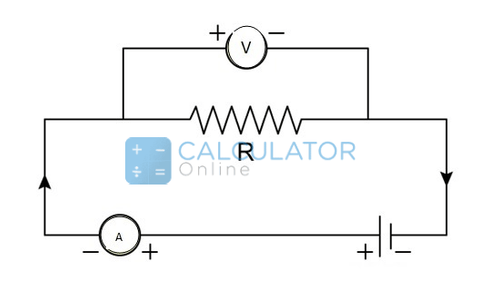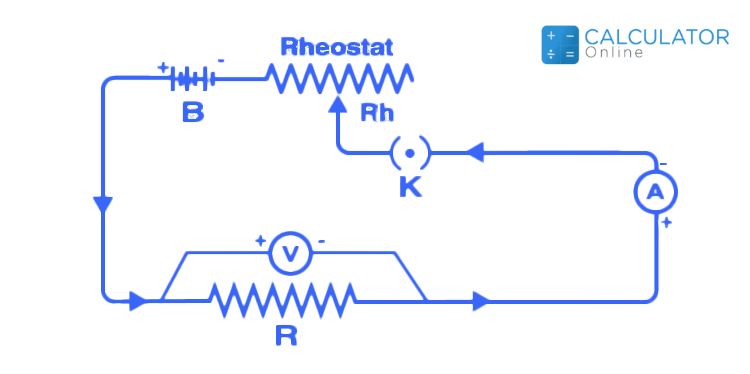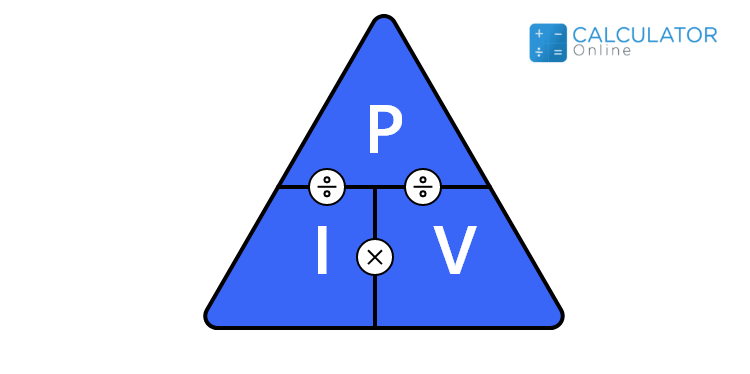• Hire UsUh Oh! It seems you’re using an Ad blocker!

We always struggled to serve you with the best online calculations, thus, there's a humble request to either disable the AD blocker or go with premium plans to use the AD-Free version for calculators.

Or# Ohm's Law Defintion & Formulas

## What is ohm's law class 10?

According to Ohm’s law definition in physics, the voltage or potential difference between two points in the circuit is proportional to the current of electricity flowing through the resistance. Usually, the three most fundamental components of electricity are voltage, current, and resistance in the circuit. Ohm’s law describes a straightforward relationship between these three variables.

### Ohms law statement:

“Ohm's law states that the applied voltage across a conductor is directly proportional to the current flowing through it, provided all physical conditions and temperature, remain constant”

It is noticeable that Ohm’s law can only hold whether the temperature and the other physical factors remain constant. In certain components, when the current increases in the circuit the temperature will also raises.

### Ohm's law formula:

Now you must think about what is the formula of ohm's law. Look at the below to know the ohm's law equation as:

V = IR

Where,
• “V” represents the voltage across the conductor
• “I” represents the current flowing through the conductor
• “R” represents the resistance provided by the conductor to the flow of current.
Also, you can find the value of voltage (V), current (I), and resistance (R) concerning the formula by using an online ohm’s law calculator

### Experimental Verification of Ohm’s Law:

Georg Simon Ohm, a German physicist (1789-1854) was the first person who verified Ohm’s law experimentally. Let’s now explain ohm's law with the help of the experiment including:
Apparatus Required:
• Resistor
• Ammeter
• Voltmeter
• Battery
• Plug Key
• Rheostat
Circuit Diagram:Procedure:
• At the initial stage, the way K is closed, and the rheostat is adjusted at the point to obtain the minimum reading in ammeter A and voltmeter V.
• The current in the given circuit is increased gradually when the sliding terminal of the rheostat moves. The current flowing in the circuit and the corresponding value of potential difference across the resistance wire are recorded during the process.
• Different sets of values voltage and current are obtained in the circuit.
• From the set of values like V and I, the ratio of V/I is calculated immediately.
• Once you calculate the ratio V/I for each set, you will notice that it is almost the same for every set. Hence, V/I = R, which is a constant value.
• Plot a graph of the current against the potential difference, it will become a straight line. This straight line represents that the current is proportional to the potential difference.
You can also use an online transformer calculator to calculate the voltage, load currents, and various losses related to the transformer.

### Ohm's law triangle:

Mathematical relationship between voltage (V, sometimes represented as U or E), resistance (R), and current (I) in an electric circuit is termed as ohm's law triangle.

You can use ohm's law triangle to remember the different ohm's law equations to solve different variables(V, I, R).
 Quantity Ohm's Law symbol Unit of measure (abbreviation) Role in circuits Voltage E Volt (V) Pressure that triggers electron flow Current I Ampere, amp (A) Rate of electron flow Resistance R Ohm (Ω) Flow inhibitor

### Solved Examples:

Now discuss the examples related to ohm’s law that are described below to clarify your concept. Let’s move!

Example #1:

If the resistance (R) of an electric iron is 100 Ω and a current of 4.2 A flows through the resistance of an electric iron. Find the voltage between two points.

Solution:

By using ohm's law formula to calculate the value of V:

V = I × R

Substituting the values in the ohm's law equation, we get

V = 4.2 A × 100 Ω = 420 V

Hence, the voltage of electric iron = 420 V

Example #2:

An EMF source of 6.0 V is connected to a resistive electrical appliance (a light bulb). An electric current flows through it is 2.0 A. Consider the conducting wires to be resistance-free. Calculate the resistance offered by the electrical appliance (a light bulb).

Solution:

To calculate the value of resistance, you can use ohm's law formula that is given as:

R = V ÷ I

Substituting the values in the ohm's law equation, we get

R = V ÷ I

R =  6 ÷ 2 A = 3 Ω
Hence, the resistance of electrical appliance =  3 Ω.

### Calculating Electrical Power Using Ohm’s Law:

Electrical power:

The rate at which energy is transferred by an electric circuit per unit time is known as electrical power.

The SI unit of power is the watt.
Generally, the electrical power can be determined using Ohm’s law by substituting the values of voltage, current, and resistance in the following formulas including:
• P = VI (when voltage and current are given)
• P = V^2/R (when both voltage and current are obtained)
• P = I^2R (when current and resistance are required)Besides, an online horsepower calculator will help you to estimate the value of manual power within a couple of seconds.

### Ohm’s Law Applications:

Generally, the main applications of Ohm’s law are given below as:
• Ohm's law is applicable to determine the value of voltage, resistance or current of an electric circuit
• Ohm’s law preserves the desired voltage drop across the electronic appliances
• Moreover, ohm’s law also used in DC ammeter and other DC shunts to transfer the current

### Limitation of ohm’s law:

There are some limitations of ohm’s law that are described below as:
• Usually, ohm's law is not applicable to unilateral networks such as diodes, transistors, and other electronic components in which current flows through one direction.
• Ohm's law is not applicable to non-linear components like capacitance, resistance, etc have a current that is not ratio to the applied voltage or current making it difficult to use Ohm’s law.